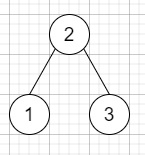# Program to find Inorder Successor of a binary search tree in C++

Suppose we have a binary search tree BST and another value of a node, we have to find the in-order successor of that node in the BST. As we all know that the successor of a node p is the node with the smallest key greater than the value of p.

So, if the input is likeAnd p = 1, then the output will be 2,

To solve this, we will follow these steps −

• Define recursive method inorderSuccessor(), this will take root and p
• if root null, then:
• return null
• if val of root <= val of p, then:
• return inorderSuccessor(right of root , p)
• Otherwise
• option := inorderSuccessor(left of root , p)
• return (if option is zero, then root, otherwise option)

Let us see the following implementation to get better understanding −

## Example

Live Demo

#include <bits/stdc++.h>
using namespace std;
class TreeNode{
public:
int val;
TreeNode *left, *right;
TreeNode(int data){
val = data;
left = NULL;
right = NULL;
}
};
class Solution {
public:
TreeNode* inorderSuccessor(TreeNode* root, TreeNode* p) {
if(!root) return NULL;
if(root->val <= p->val){
return inorderSuccessor(root->right, p);
}else{
TreeNode* option = inorderSuccessor(root->left, p);
return !option ? root : option;
}
}
};
main(){
TreeNode *root = new TreeNode(2);
root->left = new TreeNode(1);
root->right = new TreeNode(3);
TreeNode *p = root->left;
Solution ob;
cout << (ob.inorderSuccessor(root, p))->val;
}

## Input

TreeNode *root = new TreeNode(2);
root->left = new TreeNode(1);
root->right = new TreeNode(3);
1

## Output

2